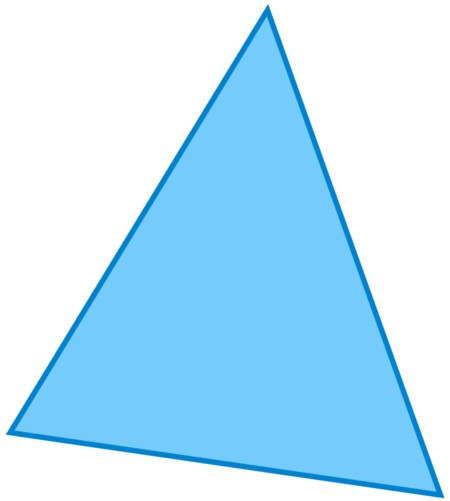## Triangle### Description

A triangle is one of the basic shapes of geometry: a polygon with three corners or vertices and three sides or edges which are line segments. A triangle with vertices A, B, and C is denoted ABC. Triangles are classified into Equilateral triangle, isosceles triangle, scalene triangle based on length of the sides. Triangles are further classified into right triangle, oblique triangle, acute triangle and obtuse triangle based on its internal angles.

### References

Triangle - For More Details